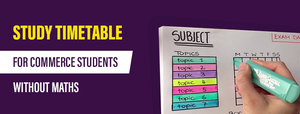Quantitative Aptitude: Banking Exams 2020 - Study24x7New to Study24X7 ?

# Quantitative Aptitude: Banking Exams 2020

Updated on 15 February 2020Banking/IRDA
Updated on 15 February 2020Preparing for banking exams in 2020?

Well, that is great! You have taken the first step towards accomplishing your dreams. However, it is very important for you to start right!Hence, in this article by study24x7.com, we are going to explain some important topics of the Quantitative Aptitude section of the banking exams.

So without wasting any time, let us get started!

First of all, we have curated a table to help you understand how much marks are assigned to the aunt section in various banking exams PO.

So basically, if you work on the quart section, this is the minimum that you can score in the exam.

Now when you have the overview of the section, we are going to explain to you the three topics that are crucial from the exam point of view.

1. Simplification: Simplification questions are often asked in the banking exam PO hence it is essential for you to understand, solve and crack such questions with ease.

To start with this topic, there are two types of simplification questions:

1. Finding the missing number
2. Determining a pattern of the given series and reducing that series into its simplest form

One example of simplification question is

(10.8×16×12)  + (3.6×56×9.2)   =?

a. 3941.35

b.3966.89

c. 3928.32

d.3649.19

e. 3645.19

Ans: c

Such types of questions are quite easy to solve if you know a couple of tricks and tips. We have made a list of such tricks so that you can become the next quant genius. So read along!

1. Learn BODMAS
2. Memorize tables up to 30
3. Learn tricks to take out square roots of numbers
4. Learn mental hacks to find cubes of number greater than 35

2. Averages: Another topic that is commonly found in the quant section of the banking exam PO is finding averages.

One example of question on averages is

If there is a class of 35 students and the average age is 14.8 years. There is another student added in the class and the average age becomes 14.9 years. What is the age of the new student?

1. 18.4
2. 16.3
3. 14.0
4. None of the above

Ans: (1)

Some tricks to solve the questions based on averages are:

1. Learn a formula’s derivation and not just the final equation
2. Learn quick tricks for taking out average of an odd and an even series
3. Practice questions of weighted average in order to crack questions in time
4. Learn mental maths

3. Percentage: Percentages questions though often asked in the quant section of the banking exam PO, they are the easiest to crack!

One example of question on averages is

A fruit seller had some oranges. He sells 30% oranges and still has 140 mangoes. Originally, he had:

A. 288 oranges

B. 300 oranges

C. 672 oranges

D. 200 oranges

Ans: D

Some tricks to solve percentage questions are:

1. Learn tricks to express percentages into fractions
2. Be very quick in taking out percentages in multiples of 10
3. Be quick with cross multiplication and equation formation

That is all from our end. We hope that now you have understood how simplification, average and percentage question are solved. For more such tricks and tips, log on to study24x7.com!

All The Best To All The RRB/NTPC 2020 Aspirants !

Write a comment...Trending ArticlesBy CBSE CLASS 8By CBSE CLASS 10By CBSE CLASS 7By K-12-Commerce
Related Posts